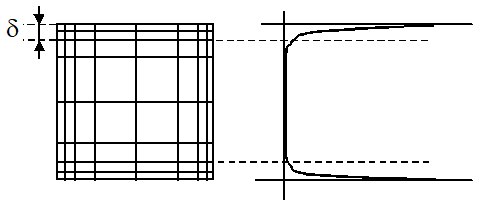# Skin effect and mesh: reminders

## Introduction

When physical phenomena are concentrated in the skin depth, a good meshing of the area can be tricky.

This document provides several strategies to mesh skin depth.

## Skin effect: reminder

The expression of the skin effect pertains to the phenomena which occur when time varying currents are flowing through a conductor.

The AC electric currents are not uniformly distributed in the conductor cross-section but are concentrated in the external layers close to the conductor surface.

This happens when the value of either the frequency (f), or the permeability (µ), or those of the electric conductivity (σ) are high.

## Skin depth: definition

The average value of “skin” depth in which the electric currents flow can be calculated using the following formula:

$\delta =\sqrt{\frac{2}{\omega \mu \sigma }}$ (for a linear, homogenous and isotropic material)

where:

ω is the current pulsation ( $\omega =2\pi f$ )

µ is the medium permeability

σ is the conductivity of medium

## Mesh of the “skin” region: rule to observe

The physical quantities of the electric current or the magnetic field are exponentially decreasing in the skin depth.

In order to have an accurate evaluation of the physical quantities in the skin depth at the surface of the conductor: it is necessary to have at least two layers of elements (of second order) in the thickness of this region.
A mesh example of the skin depth region is presented in the figure:## Economical mesh with rectangular elements (or hexahedron)

To mesh the skin depth it may be judicious to use rectangular type elements (or hexahedron in 3D) in the skin depth zone.

Indeed, with the physical phenomena concentrated in a low thickness depth, it is possible to significantly distort the elements in order that they should get adapted to the physical requirements of the problem.

It allows reducing a lot the number of elements in comparison with a triangular mesh (or tetrahedron). This type of mesh is therefore very economical in number of nodes, especially in 3D.

The rest of the study domain can be meshed by triangular elements.